# SVM---通俗易懂图解高斯核函数及实现

$min\frac1m[\sum_{i=1}^m{y^{(i)}(-log{h_\theta(x^{(i)})}) + (1-y^{(i)})((-log(1-h_\theta(x^{(i)})))}]$+$\frac{\lambda}{2m}\sum_{j=1}^n{\theta_j^2}$

SVM的代价函数只是稍微进行修改：

$minC[\sum_{i=1}^m{y^{(i)}cost_1(\theta^Tx^{(i)}))})$+ $(1-y^{(i)})cost_0(\theta^Tx^{(i)})$+$\frac12\sum_{j=1}^n{\theta_j^2}$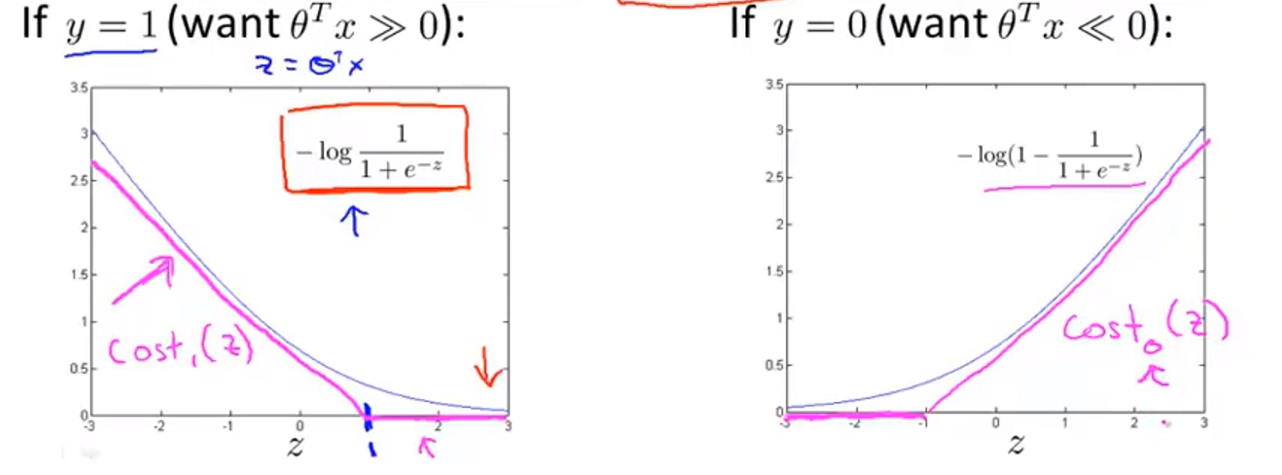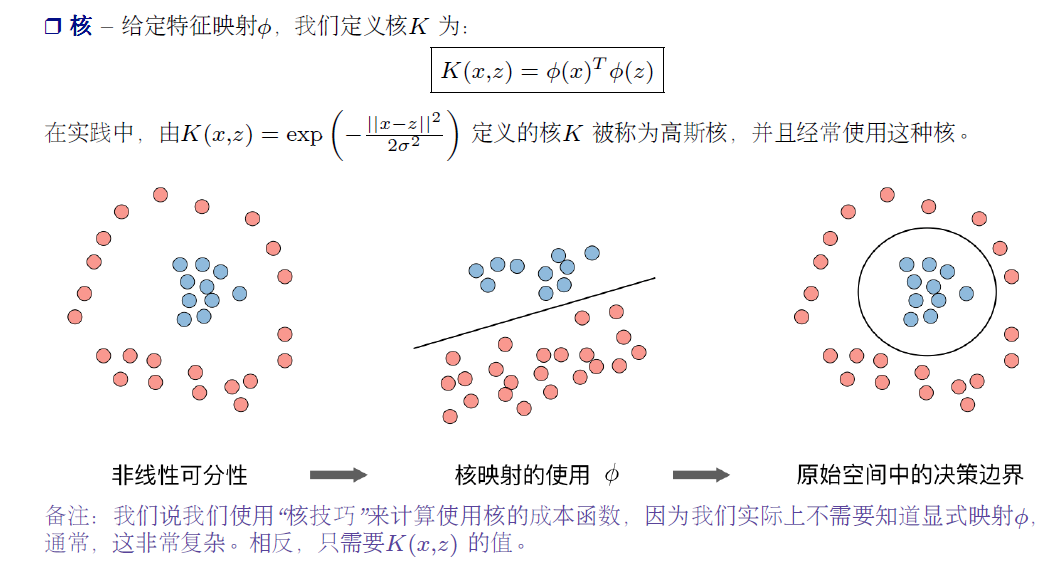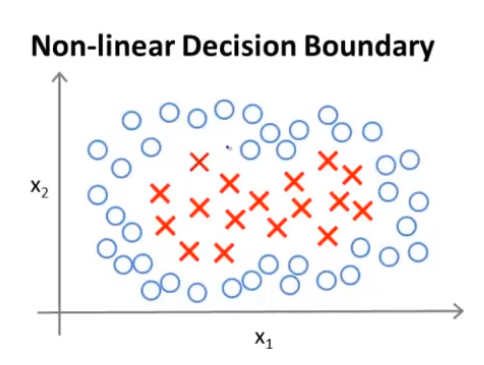当$\theta^Tx^{(i)} >= 1$$y=1$为图中红色X
否则，$y=0$为图中蓝色O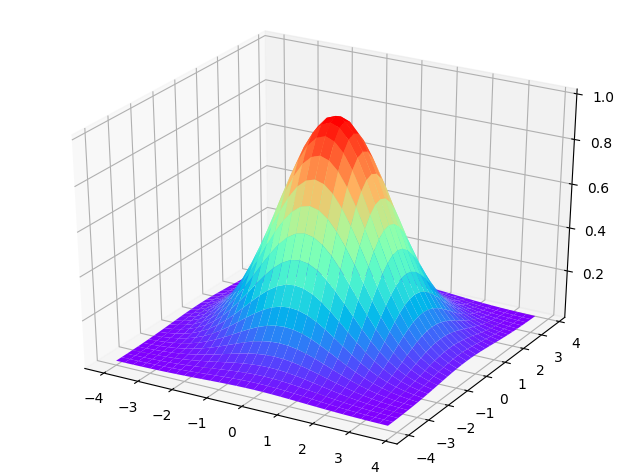$y = e^{(-{\frac{(x_1-\mu)^2+(x_2-\mu)^2}{2\sigma^2}})}$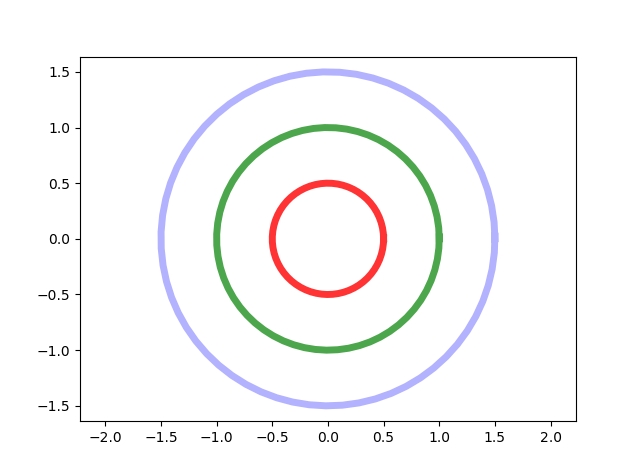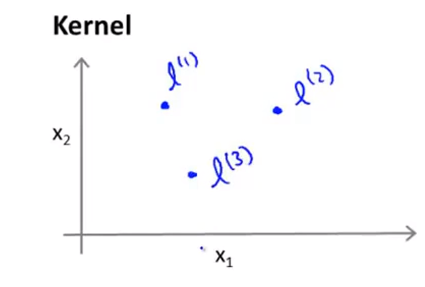$\theta^Tf(x^{(i)}) >= 1$$y=1$为图中红色X

# 高斯核的实现方法
def RBF(x, L, sigma):
'''
x: 待分类的点的坐标
L: 某些中心点，通过计算x到这些L的距离的和来判断类别
'''
return np.exp(-(np.sum((x - L) ** 2)) / (2 * sigma**2))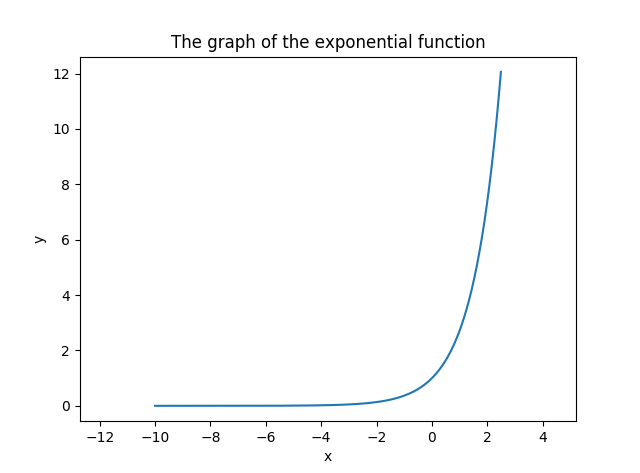Note: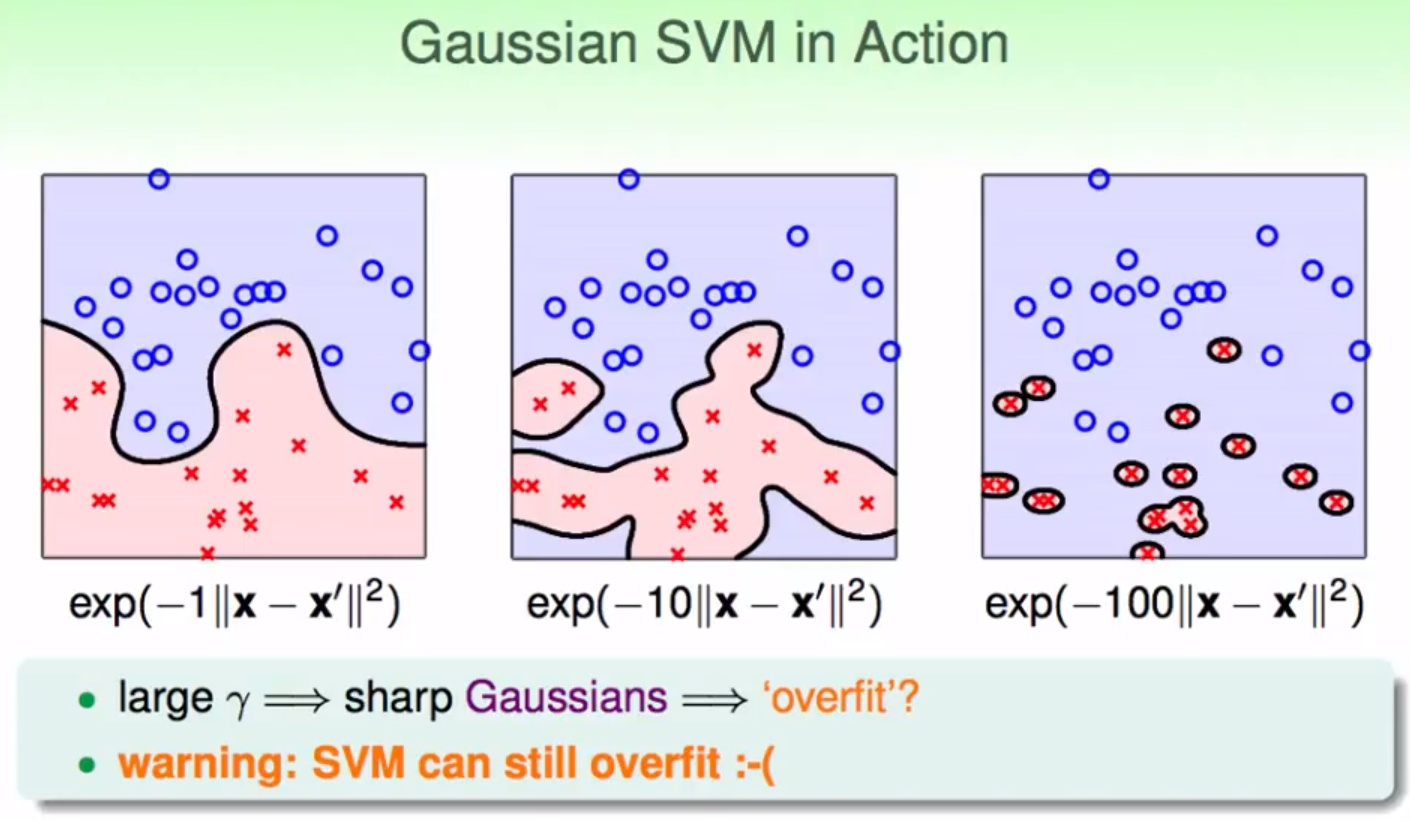©️2019 CSDN 皮肤主题: 精致技术 设计师: CSDN官方博客